HashMap线程不安全的体现

1.jdk1.7中的HashMap

1 public class HashMapTest {
2
3     public static void main(String[] args) {
14     }
15 }
16
18     private static AtomicInteger ai = new AtomicInteger();
19     private static Map<Integer, Integer> map = new HashMap<>();
20
21     @Override
22     public void run() {
23         while (ai.get() < 1000000) {
24             map.put(ai.get(), ai.get());
25             ai.incrementAndGet();
26         }
27     }
28 }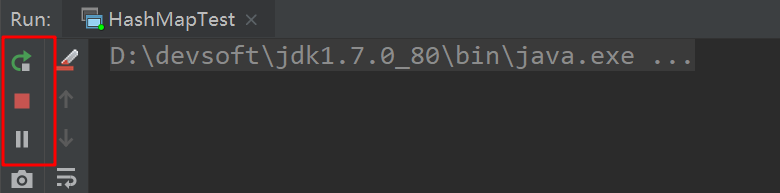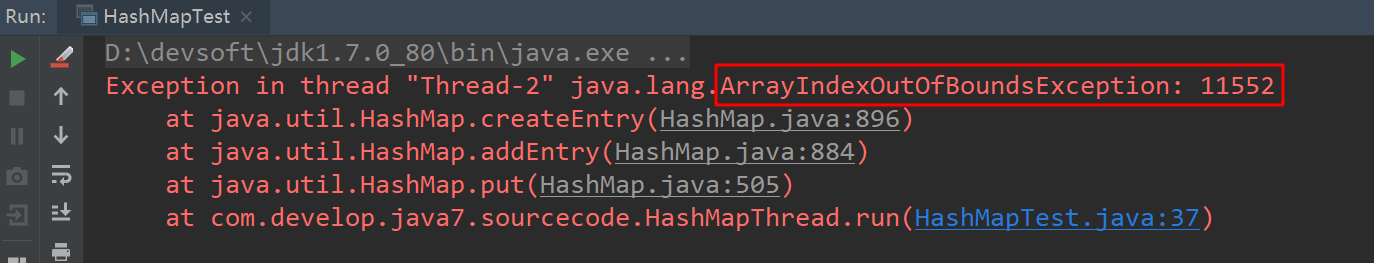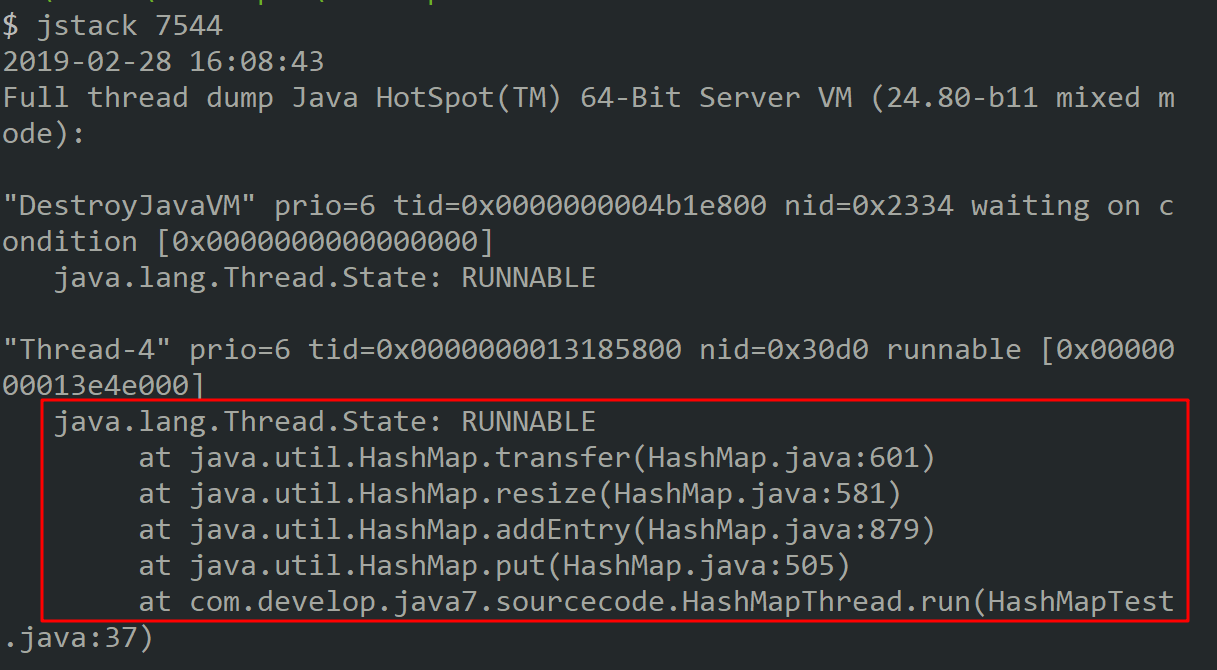1    void transfer(Entry[] newTable, boolean rehash) {
2         int newCapacity = newTable.length;
3         for (Entry<K,V> e : table) {
4             while(null != e) {
5                 Entry<K,V> next = e.next;
6                 if (rehash) {
7                     e.hash = null == e.key ? 0 : hash(e.key);
8                 }
9                 int i = indexFor(e.hash, newCapacity);
10                 e.next = newTable[i];
11                 newTable[i] = e;
12                 e = next;
13             }
14         }
15     }

1.1 扩容造成死循环分析过程

#1.hash算法为简单的用key mod链表的大小。

#2.最开始hash表size=2，key=3,7,5，则都在table中。

#3.然后进行resize，使size变成4。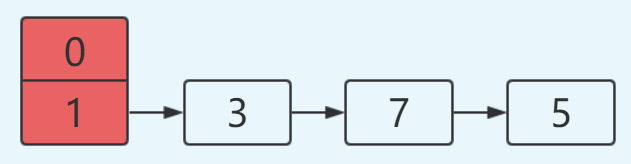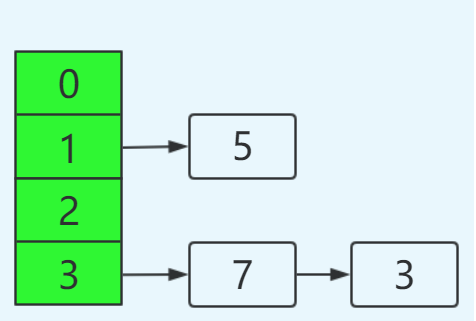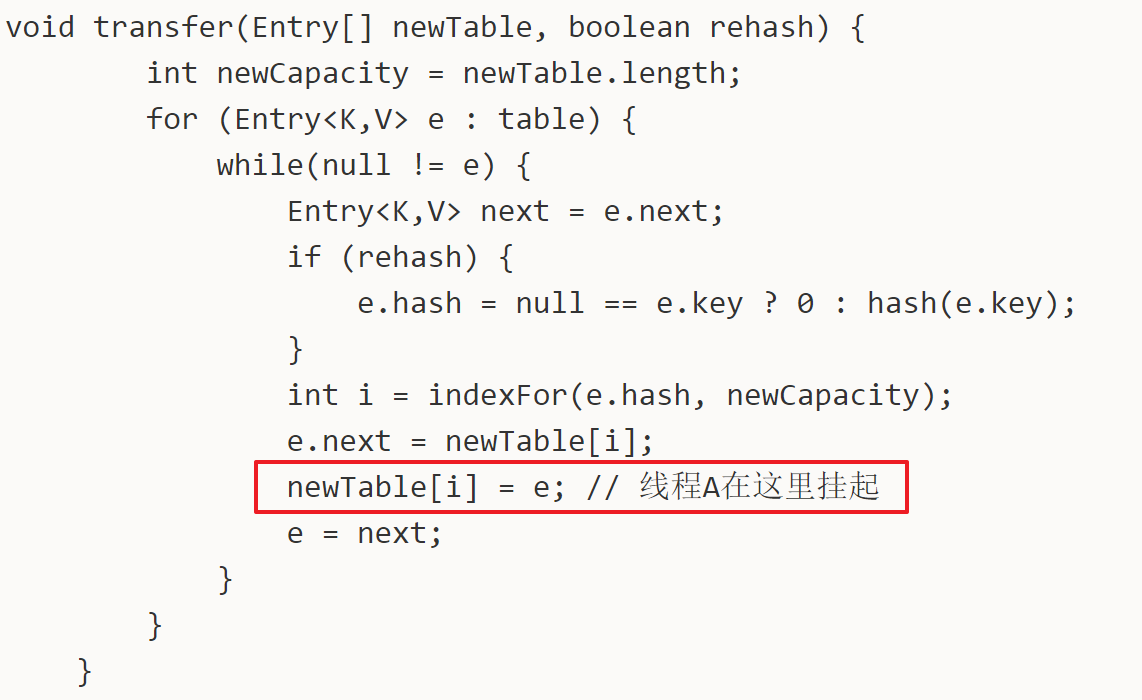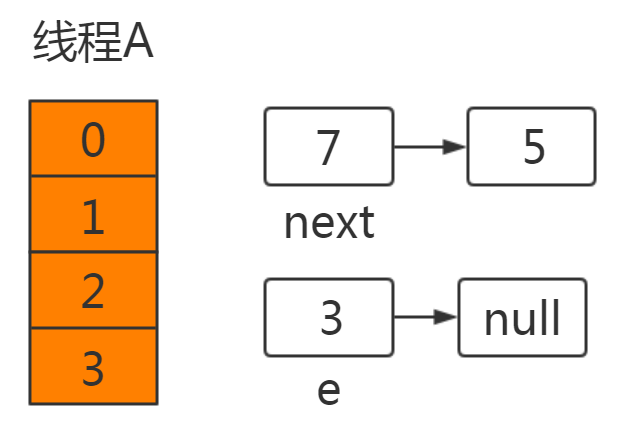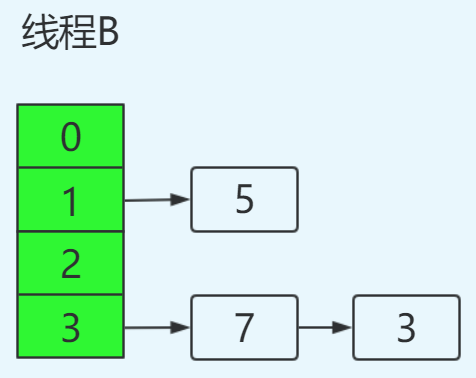newTable=e ----> newTable=3
e=next ----> e=7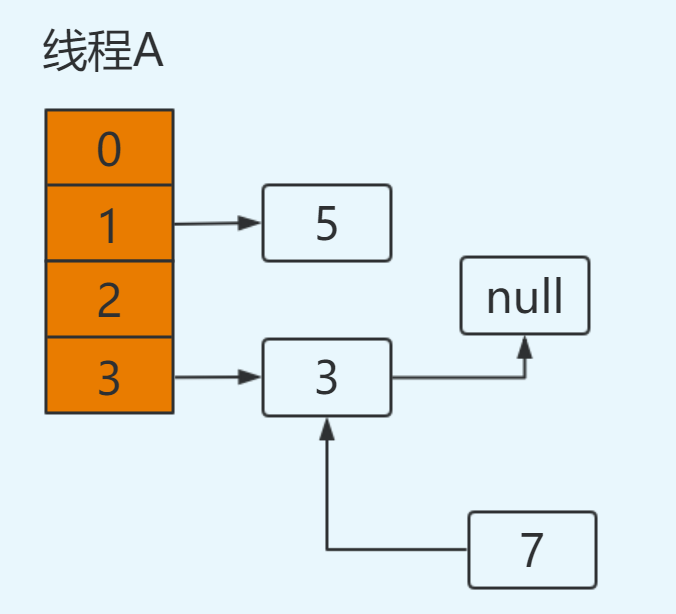e=7
next=e.next ----> next=3从主存中取值】
e.next=newTable ----> e.next=3从主存中取值】
newTable=e ----> newTable=7
e=next ----> e=3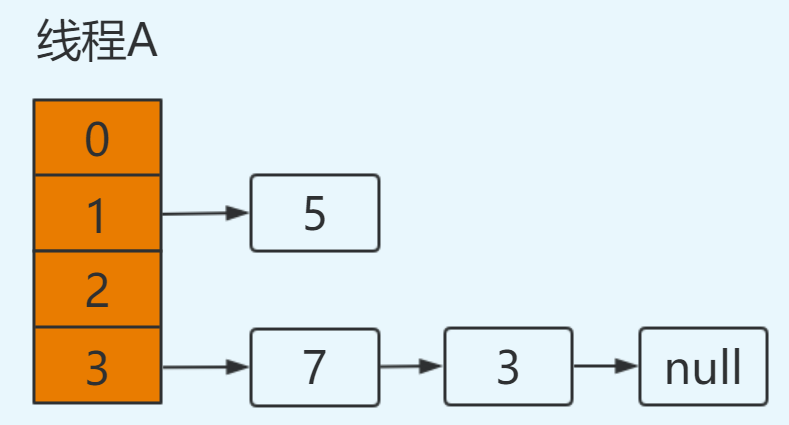e=3
next=e.next ----> next=null
e.next=newTable ----> e.next=7 即：3.next=7
newTable=e ----> newTable=3
e=next ----> e=null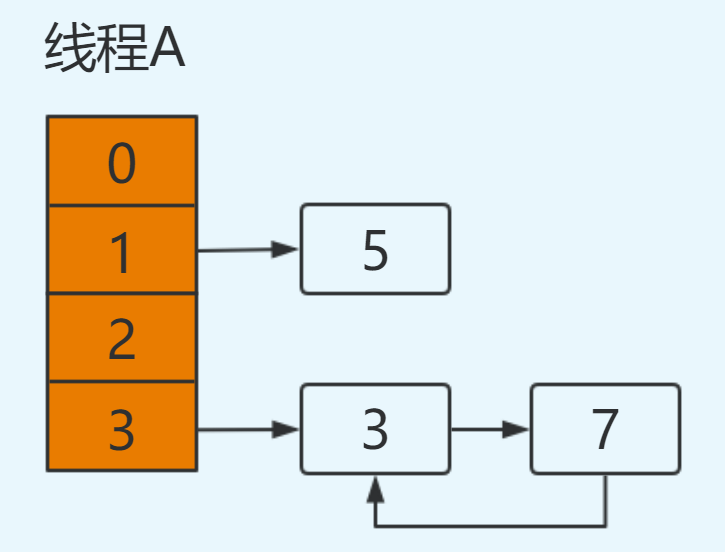1.2 扩容造成数据丢失分析过程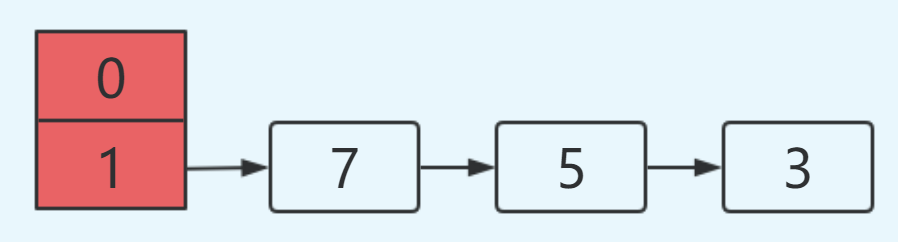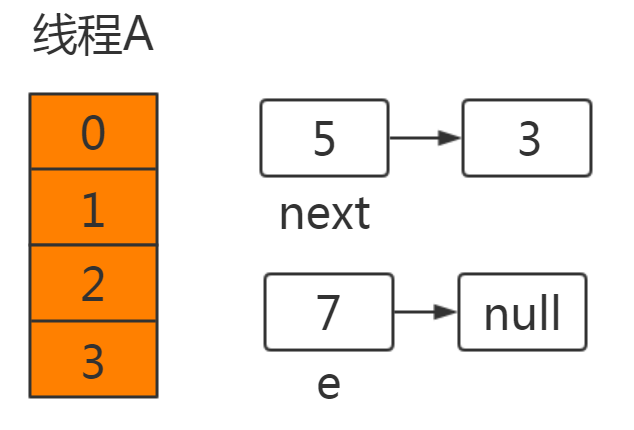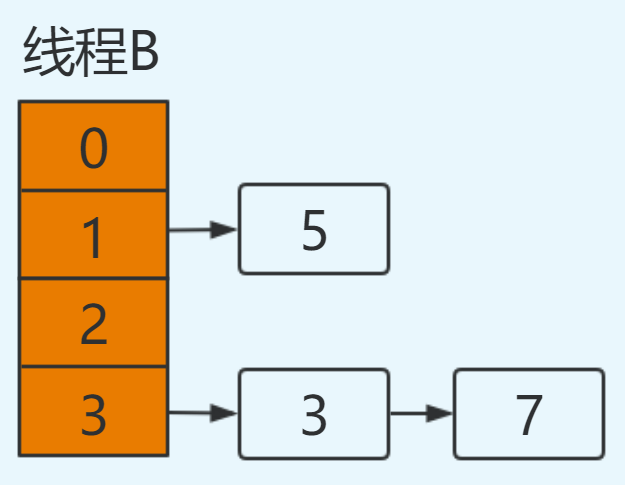e=5
next=e.next ----> next=null，从主存中取值
e.next=newTable ----> e.next=5，从主存中取值
newTable=e ----> newTable=5
e=next ----> e=null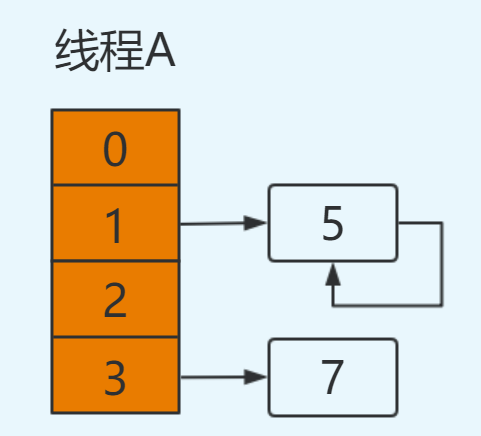2.jdk1.8中HashMap

1  final V putVal(int hash, K key, V value, boolean onlyIfAbsent,
2                    boolean evict) {
3         Node<K,V>[] tab; Node<K,V> p; int n, i;
4         if ((tab = table) == null || (n = tab.length) == 0)
5             n = (tab = resize()).length;
6         if ((p = tab[i = (n - 1) & hash]) == null) // 如果没有hash碰撞则直接插入元素
7             tab[i] = newNode(hash, key, value, null);
8         else {
9             Node<K,V> e; K k;
10             if (p.hash == hash &&
11                 ((k = p.key) == key || (key != null && key.equals(k))))
12                 e = p;
13             else if (p instanceof TreeNode)
14                 e = ((TreeNode<K,V>)p).putTreeVal(this, tab, hash, key, value);
15             else {
16                 for (int binCount = 0; ; ++binCount) {
17                     if ((e = p.next) == null) {
18                         p.next = newNode(hash, key, value, null);
19                         if (binCount >= TREEIFY_THRESHOLD - 1) // -1 for 1st
20                             treeifyBin(tab, hash);
21                         break;
22                     }
23                     if (e.hash == hash &&
24                         ((k = e.key) == key || (key != null && key.equals(k))))
25                         break;
26                     p = e;
27                 }
28             }
29             if (e != null) { // existing mapping for key
30                 V oldValue = e.value;
31                 if (!onlyIfAbsent || oldValue == null)
32                     e.value = value;
33                 afterNodeAccess(e);
34                 return oldValue;
35             }
36         }
37         ++modCount;
38         if (++size > threshold)
39             resize();
40         afterNodeInsertion(evict);
41         return null;
42     }

总结

#1.在jdk1.7中，在多线程环境下，扩容时会造成环形链或数据丢失。

#2.在jdk1.8中，在多线程环境下，会发生数据覆盖的情况。

by Shawn Chen，2019.03.02，上午。

=========================================================

posted @ 2019-03-02 10:52  developer_chan  阅读(28285)  评论(3编辑  收藏  举报